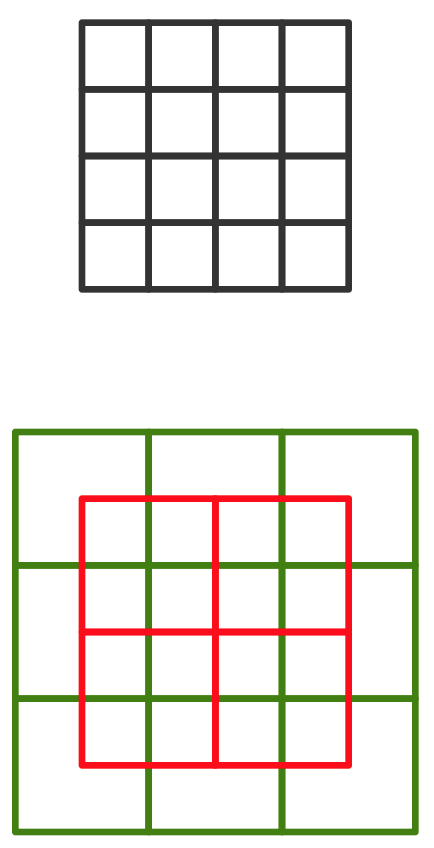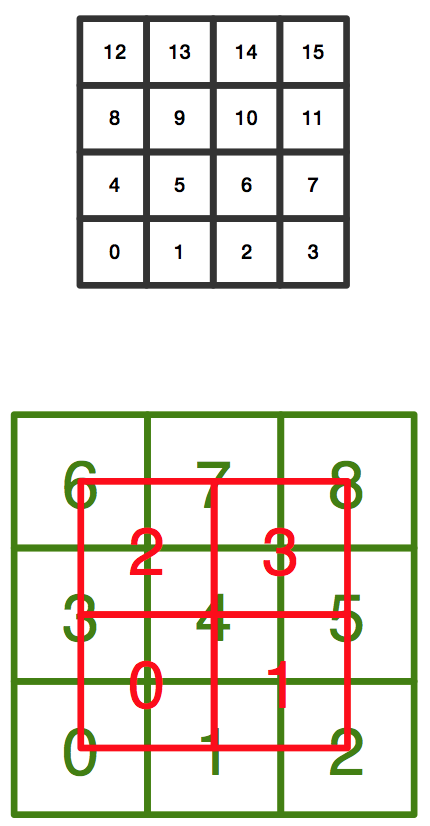## Thursday, 5 March 2015

### Converting numeric data to coarse codedense vs coarse codefor 16 bits number in dense and equivalent 13 bits coarse code.
OK, suppose you have a single variable that represents the current value of a sensor. A network usage traffic, the heat bitrate of a human and ... at each time when you read the sensor value you just get a numeric number, suppose it is an integer number in the interval [0,65535] . If you want to store and process it in a dense format you need 16 bits (or neurons) to show the sensor output in dense binary format.

Let us get back to the previous post and represent the 16 bits number as a 4 × 4 square, like the picture. So with this 16 bits memory page, we can show numbers from 0 to 65,535. As you may remember if we want to use coarse coding to represent these 16 bits we need a total of 13 bits, as shown in the picture. Note that as the size of the pages get larger and memory grows the efficiency of gets better and better. But for now, forget about the efficiency, just try to understand how it works.

Where is the problem?
The problem is our storage systems or models are built/designed to store dense binary data. Even our imaginations digest and understand these kinds of storages better. So we have no choice other than figuring out a way to represent this 13 bits in an ordinary dense binary system. This may also cost us some coding and decoding procedures too.16 / 13 ~ 123% performance
But the answer is not that hard
Look at the picture again, this time with bit numbers. As you see we have bits from 0 to 15 to represent a number in dense binary format. We can have it in 13 bits coarse model as two separate 8 and 4 bits dense format. Now if you have these two number you can have the sensor value like when you have 16 bits value.

For example for number 34,953 we have:

(34,93)10  = (1 0 0 0 1 0 0 0 1 0 0 0 1 0 0 1)2

Or in our page form as:

[
[0 0 0 1],
[0 0 0 1],
[0 0 0 1],
[1 0 0 1]
]

To represent it in our 13 bits coarse code we can have it like these:

[
[0 1],
[1 1]
]

and

[
[0 0 1],
[0 0 1],
[1 0 1]
]

These two numbers are 11 and 293, wonderful, no? The following picture helps you to find out why. (Just consider this representation in flat plane can't show all possible values. We need to have these two coordinates overlapped in more than neighbor cells, we will talk about it later.)A model to convert coarse code to dense binary storage

Do you want better performance?
Just look at the bellow picture, in which we can represent a 49 bits number in just 27 bits!? The question is why it is like this? How does it work? Can we make a computer work in this way?49 / 27 ~ 181% performance